# What Is a Tensor?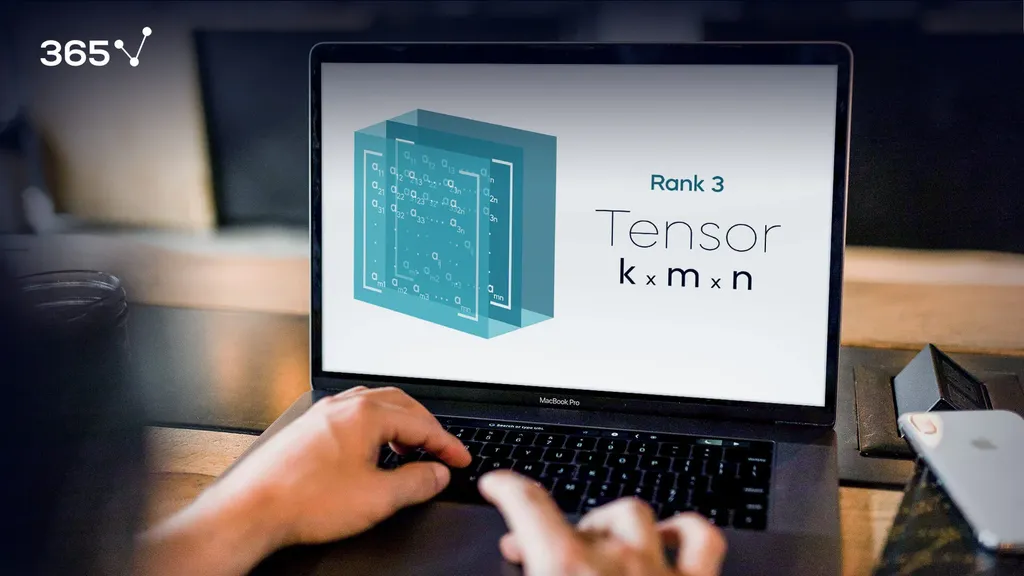Tensors have been around for nearly 200 years. In fact, the first use of the word ‘tensor’ was introduced by William Hamilton. Interestingly, the meaning of this word had little to do with what we call tensors from 1898 until today.

How did tensors become important you may ask? Well, not without the help of one of the biggest names in science – Albert Einstein! Einstein developed and formulated the whole theory of ‘general relativity’ entirely in the language of tensors. Having done that, Einstein, while not a big fan of tensors himself, popularized tensor calculus to more than anyone else could ever have.

Nowadays, we can argue that the word ‘tensor’ is still a bit ‘underground’. You won’t hear it in high school. In fact, your Math teacher may have never heard of it. However, state-of-the-art machine learning frameworks are doubling down on tensors. The most prominent example being Google’s TensorFlow.

## What is a tensor in Layman’s terms?

The mathematical concept of a tensor could be broadly explained in this way.

A scalar has the lowest dimensionality and is always 1x1. It can be thought of as a vector of length 1, or a 1x1 matrix.

It is followed by a vector, where each element of that vector is a scalar. The dimensions of a vector are nothing but Mx1 or 1xM matrices.

Okay.

Then we have matrices, which are nothing more than a collection of vectors. The dimensions of a matrix are MxN. In other words, a matrix is a collection of n vectors of dimensions m by 1. Or, m vectors of dimensions n by 1.

Furthermore, since scalars make up vectors, you can also think of a matrix as a collection of scalars, too.

Now, a tensor is the most general concept.

Scalars, vectors, and matrices are all tensors of ranks 0, 1, and 2, respectively. Tensors are simply a generalization of the concepts we have seen so far.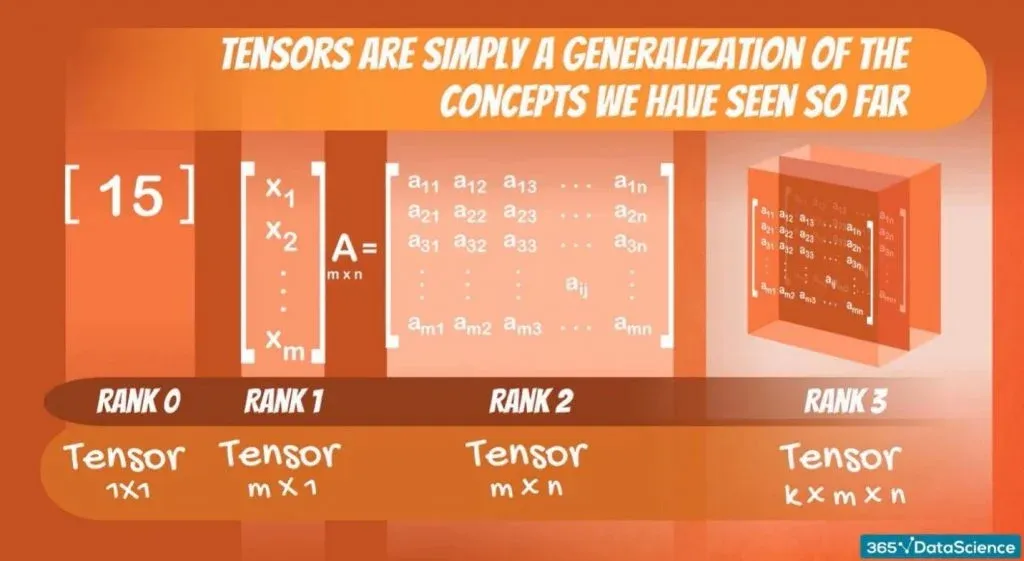An object we haven’t seen is a tensor of rank 3. Its dimensions could be signified by k,m, and n, making it a KxMxN object. Such an object can be thought of as a collection of matrices.

## How do you ‘code’ a tensor?

Let’s look at that in the context of Python.

In terms of programming, a tensor is no different than a NumPy ndarray. And in fact, tensors can be stored in ndarrays and that’s how we often deal with the issue.

Let’s create a tensor out of two matrices.

Our first matrix m1 will be a matrix with two vectors: [5, 12, 6] and [-3, 0, 14].

The matrix m2 will be a different one with the elements: [9, 8, 7] and [1, 3, -5].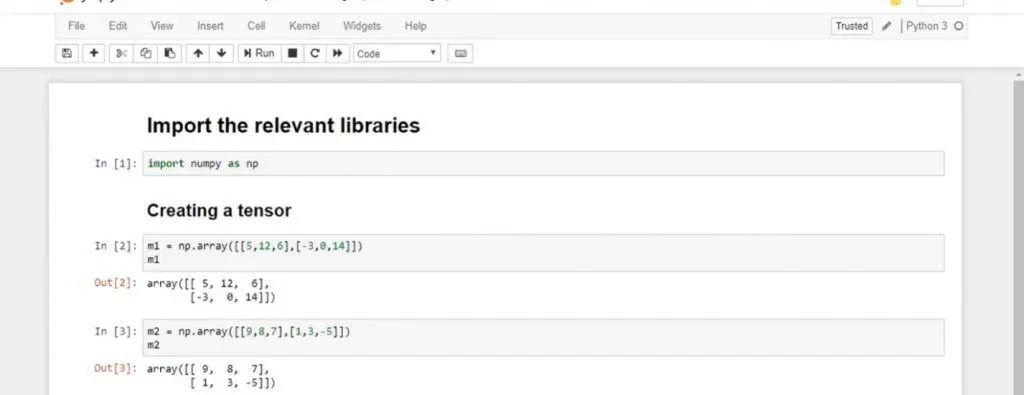Now, let’s create an array, T, with two elements: m1 and m2.

After printing T, we realize that it contains both matrices.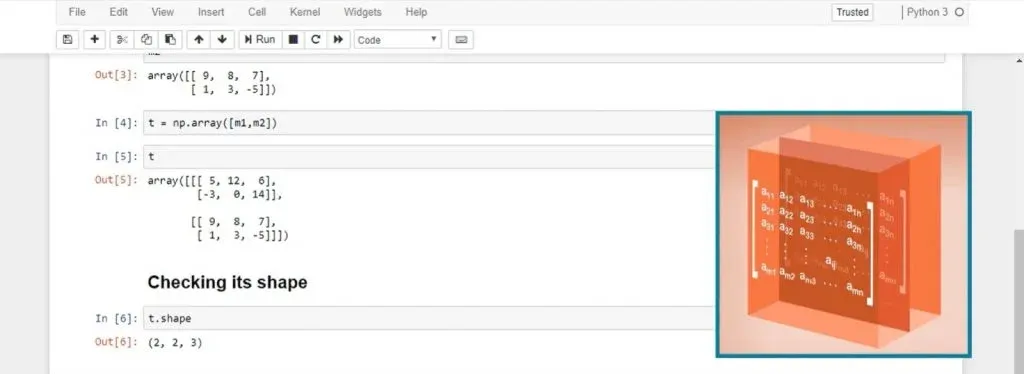It is a 2x2x3 object. It contains two matrices, 2x3 each.

Alright.

If we want to manually create the same tensor, we would need to write this line of code.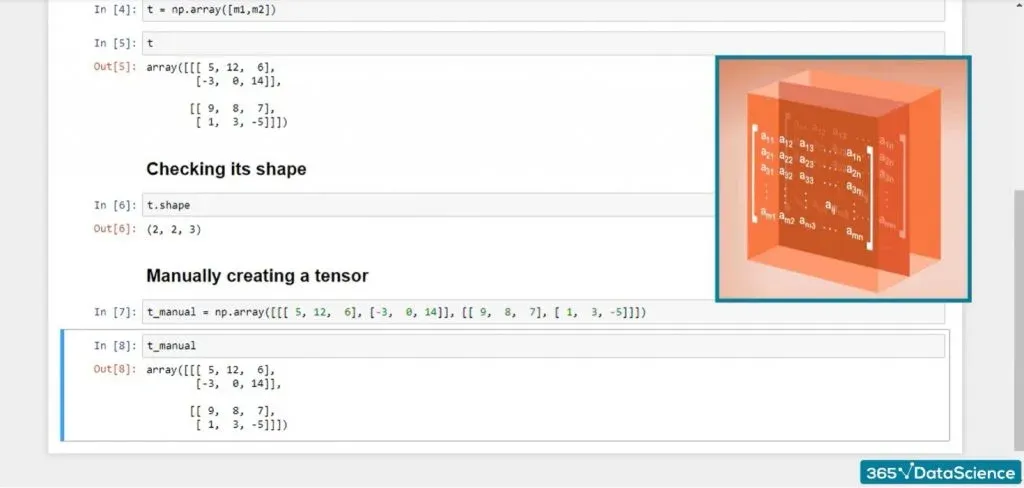As you can imagine, tensors with lots of elements are very hard to manually create. Not only because there are many elements, but also because of those confusing brackets.

Usually, we would load, transform, and preprocess the data to get tensors. However, it is always good to have the theoretical background.

## Why are tensors useful in TensorFlow?

After this short intro to tensors, a question still remains – why TensorFlow is called like that and why does this framework need tensors at all.

First of all, Einstein has successfully proven that tensors are useful.

Second, in machine learning, we often explain a single object with several dimensions. For instance, a photo is described by pixels. Each pixel has intensity, position, and depth (color). If we are talking about a 3D movie experience, a pixel could be perceived in a different way from each of our eyes. That’s where tensors come in handy – no matter the number of additional attributes we want to add to describe an object, we can simply add an extra dimension in our tensor. This makes them extremely scalable, too.

Finally, we’ve got different frameworks and programming languages. For instance, R is famously a vector-oriented programming language. This means that the lowest unit is not an integer or a float; instead, it is a vector. In the same way, TensorFlow works with tensors. This not only optimizes the CPU usage, but also allows us to employ GPUs to make calculations. What’s more, in 2016 Google developed TPUs (tensor processing units). These are processors, which consider a ‘tensor’ a building block for a calculation and not 0s and 1s as does a CPU, making calculations exponentially faster.

So, tensors are a great addition to our toolkit, if we are looking to expand into machine and deep learning. If you want to get into that, you can learn more in our Deep Learning with TensorFlow 2 and Convolutional Neural Networks with TensorFlow in Python courses.

## Ready to take the next step towards a data science career?

Check out the complete Data Science Program today. Start with the fundamentals with our Statistics, Maths, and Excel courses. Build up a step-by-step experience with SQL, Python, R, Power BI, and Tableau. And upgrade your skillset with Machine Learning, Deep Learning, Credit Risk Modeling, Time Series Analysis, and Customer Analytics in Python. Still not sure you want to turn your interest in data science into a career? You can explore the curriculum or sign up 12 hours of beginner to advanced video content for free by clicking on the button below.

Learn data science with industry expertsStart your career